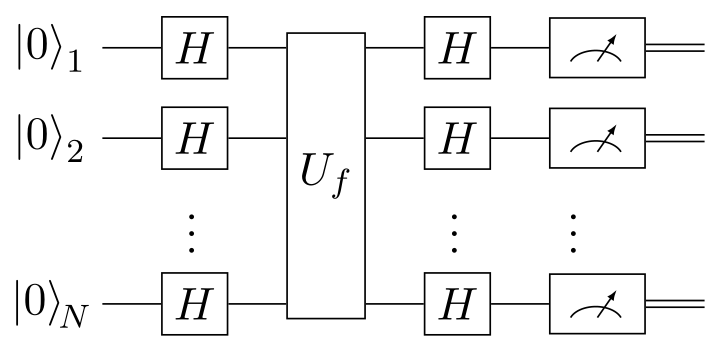Categories: Algorithms, Quantum information.

# Bernstein-Vazirani algorithm

In quantum information, the Bernstein-Vazirani algorithm proves the supremacy of quantum computers over classical deterministic or probabilistic computers. It is extremely similar to the Deutsch-Jozsa algorithm, and even uses the same circuit.

It solves a very artificial problem: we are given a “black box” function $$f(x)$$ that takes an $$N$$-bit $$x$$ and returns a single bit, which we are promised is the lowest bit of the bitwise dot product of $$x$$ with an unknown $$N$$-bit string $$s$$:

\begin{aligned} f(x) = s \cdot x \:\:(\bmod 2) = (s_1 x_1 + s_2 x_2 + \:...\: + s_N x_N) \:\:(\bmod 2) \end{aligned}

The goal is to find $$s$$. To solve this problem, a classical computer would need to call $$f(x)$$ exactly $$N$$ times with $$x = 2^n$$ for $$n \in \{ 0, ..., N \!-\! 1\}$$. However, the Bernstein-Vazirani algorithm allows a quantum computer to do it with only a single query. It uses the following circuit:Where $$U_f$$ is a phase oracle, whose action is defined as follows, where $$\ket{x} = \ket{x_1} \cdots \ket{x_N}$$:

\begin{aligned} \ket{x} \quad \to \boxed{U_f} \to \quad (-1)^{f(x)} \ket{x} = (-1)^{s \cdot x} \ket{x} \end{aligned}

That is, it introduces a phase flip based on the value of $$f(x)$$. For an example implementation of such an oracle, see the Deutsch-Jozsa algorithm: its circuit is identical to this one, but describes $$U_f$$ in a different (but equivalent) way.

Starting from the state $$\ket{0}^{\otimes N}$$, applying the Hadamard gate $$H$$ to all qubits yields:

\begin{aligned} \ket{0}^{\otimes N} \quad \to \boxed{H^{\otimes N}} \to \quad \ket{+}^{\otimes N} = \frac{1}{\sqrt{2^N}} \sum_{x = 0}^{2^N - 1} \ket{x} \end{aligned}

This is an equal superposition of all candidates $$\ket{x}$$, which we feed to the oracle:

\begin{aligned} \frac{1}{\sqrt{2^N}} \sum_{x = 0}^{2^N - 1} \ket{x} \quad \to \boxed{U_f} \to \quad \frac{1}{\sqrt{2^N}} \sum_{x = 0}^{2^N - 1} (-1)^{s \cdot x} \ket{x} \end{aligned}

Then, thanks to the definition of the Hadamard transform, a final set of $$H$$-gates leads us to:

\begin{aligned} \frac{1}{\sqrt{2^N}} \sum_{x = 0}^{2^N - 1} (-1)^{s \cdot x} \ket{x} \quad \to \boxed{H^{\otimes N}} \to \quad \ket{s} = \ket{s_1} \cdots \ket{s_N} \end{aligned}

Which, upon measurement, gives us the desired binary representation of $$s$$. For comparison, the Deutsch-Jozsa algorithm only cares whether $$s = 0$$ or $$s \neq 0$$, whereas this algorithm is interested in the exact value of $$s$$.

1. J.S. Neergaard-Nielsen, Quantum information: lectures notes, 2021, unpublished.
2. S. Aaronson, Introduction to quantum information science: lecture notes, 2018, unpublished.